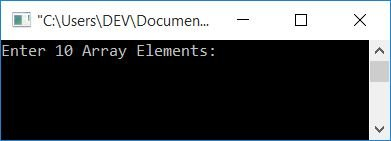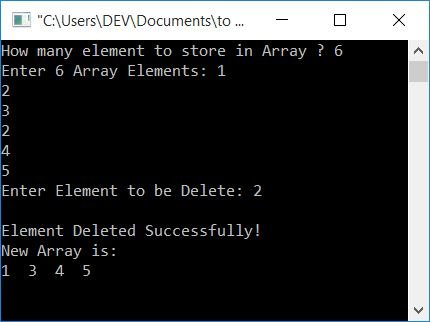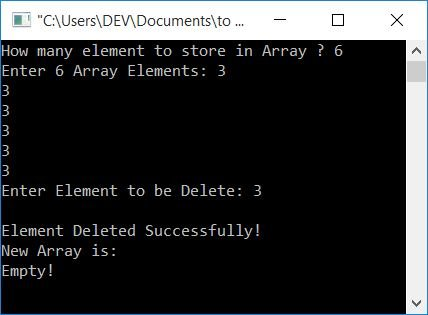# C Program to Delete an Element from an Array

In this article, you will learn and get code for deleting an element from an array given by the user (at run-time).

## Delete an element from an array

Let's first create a program that deletes the desired element from the list and prints the message "The element is deleted."

```#include<stdio.h>
#include<conio.h>
int main()
{
int arr, del, i, j, found=0, size;
printf("Enter 10 Array Elements: ");
for(i=0; i<10; i++)
scanf("%d", &arr[i]);
printf("Enter Element to be Delete: ");
scanf("%d", &del);
size = 10;
for(i=0; i<size; i++)
{
if(arr[i]==del)
{
for(j=i; j<(size-1); j++)
arr[j] = arr[j+1];
found=1;
i--;
size--;
}
}
if(found==0)
else
printf("\nElement Deleted Successfully!");
getch();
return 0;
}```

This program was built and runs under the Code::Blocks IDE. Here is its sample run:Now supply any 10 numbers and then an element that has to be deleted from the given array. Here is its sample run:#### Program Explained

• Here all the 10 array elements get stored in arr[] in a way that
• The first number goes to arr.
• The second number goes to arr.
• and so on.
• Create a for loop that runs 10 times to compare the given element with each and every element available in the list.
• If the given element (to be deleted) gets matched with a number at any index, say 4,
• Then move all the elements in index one back from here. Like
• The element at index 5 is moved to index 4.
• The element at index 6 is moved to index 5.
• and so on.
• Initialize 1 to found just to see if this if block is executed or not.If the command is executed, the given number is found in the list. Otherwise, it does not discover
• Then decrement the value of i to check from one index back (from where it starts before entering the inner for loop).
• Because the new element at index 4 may once again equal the number.
• Also, decrement the size value because one element is deleted.
• And continue the same process until all the elements of the given array have been compared.
• Check to see if the value of found is 0 or not.
• If its value is 0, then no match gets found. So print a message saying the element does not exist.
• Otherwise, if its value is not zero, it means its value is 1, and the value gets printed like an element that gets deleted.
• Don't forget to initialize 0 to "found" at the start of the program.

As you can see, the element gets deleted. But to clarify, whether it actually deletes the element or not, Then we have to print the array after removing the element. Let's create a program to solve this problem.

## Delete an Element and Print a New Array

Here is the complete version of the above program.

```#include<stdio.h>
#include<conio.h>
int main()
{
int arr, size, del, i, j, found=0;
printf("How many element to store in Array ? ");
scanf("%d", &size);
printf("Enter %d Array Elements: ", size);
for(i=0; i<size; i++)
scanf("%d", &arr[i]);
printf("Enter Element to be Delete: ");
scanf("%d", &del);
for(i=0; i<size; i++)
{
if(arr[i]==del)
{
for(j=i; j<(size-1); j++)
arr[j] = arr[j+1];
found=1;
i--;
size--;
}
}
if(found==0)
else
{
printf("\nElement Deleted Successfully!");
printf("\nNew Array is:\n");
if(size==0)
printf("Empty!");
else
{
for(i=0; i<size; i++)
printf("%d  ", arr[i]);
}
}
getch();
return 0;
}```

Let's take a look at its two sample runs. The first one is:As shown in the preceding screenshot, element 2 is found twice in the list and is deleted. Let's put it to the test: what if all of the array's elements are the same? And the user enters the same element to be deleted, as shown here:As you can see, since all the elements of the array are the same, that is 3. And the user enters 3 to be deleted; therefore, after deleting 3, the array becomes empty.

#### The same program in different languages

C Quiz

« Previous Program Next Program »